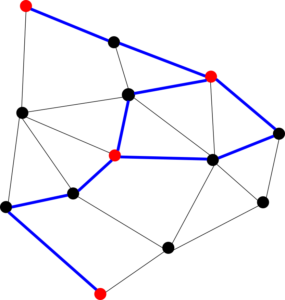models and algorithms: from the discrete to the continuous

# Discrete mathematics and algorithms

There is a large set of interactions in discrete mathematics and algorithms. We detail three topics that are particularly ripe, and then give an outlook of other directions. Contributors of the Bézout Labex on these questions are among others Éric Colin de Verdière, Jean-François Delmas, Matthieu Fradelizi, Alfredo Hubard, Frédéric Meunier, Paul-Marie Samson, and Pierre-André Zitt.

Discrete optimizationOur expertise in discrete optimization ranges from its effective application to real-world problems (e.g., operations research, bioinformatics) to optimization methodology (e.g., FPT, local search, fixed-genus graph algorithms) and the analysis of underlying combinatorial structures (e.g., hypergraph coloring or epsilon-nets). Theoreticians focus on methods whereas practitioners focus on problems and this, as usual in the field, drastically hinders interactions.  An applied topic that has been investigated in the past and which will be probably again addressed in the future is that of the optimization of new urban mobility systems. How to rebalance the stations for bike sharing is an example of a concrete question that comes under this topic. This question, also related to smart cities, is wide open and presents various challenges (routing, inventory, real-time pricing, etc.). More systematically, the monitoring and analysis of urban networks requires a measurement system that builds on, and integrates, the multiple elements composing the city, from individual players to infrastructure to social networks.  These are active research topics at CERMICS and LIGM, which contribute as well to the smart cities area.

We also focus on more theoretical topics, for example T-joins, which are widely used in optimization on undirected graphs (e.g., shortest paths with negative weights, matching polytope, postman problems). T-joins for planar graphs enjoy additional properties (cf. Seymour’s theorem on planar integer multiflow) and we have started to explore extensions to graphs of higher genus. Other specific problems include the structure of independent sets in graphs or hypergraphs.

Limits of combinatorial structuresGraphons are mathematical structures developed over the past 15 years by Lovász, Razborov and others to describe the structure of large graphs in probabilistic, combinatorial, and algebraic terms. These equivalent formalisms offer a powerful discrete/continuous gateway that was instrumental to solve long-standing extremal problems (e.g., relate the triangle density to the edge density in large graphs). A group (CERMICS and LAMA) studies how graphons approximate large networks in a statistical sense. Another group (LIGM) applies ideas from graphons to other combinatorial structures (e.g., oriented matroids or order types) encoding extremal problems in combinatorial geometry (e.g., Sylvester’s problem or random generation).

These two directions abund with open questions. On the one hand, the central limit theorems for the convergence of random graphs to their graphon, to allow to analyze statistically the likelihood of a given graph to sample a given graphon. On the other hand, graphon-type representations of combinatorial structures arising from geometry often reflect intricate internal constraints that remain to elucidate.

Random discrete structuresRandom discrete structures are often studied through related continuous models. For instance, as pioneered by Bárány and Larman, estimates on invariants of random polytopes rely on volumes of floating bodies, and the study of discrete Galton-Watson trees relies on the eponymous continuous branching processes.  Going in the other direction, it is customary to explore continuous phenomena mechanically, via discretizations. This raises many difficult issues, among which the enumeration or the random generation of discrete structures.

We generalize the Bárány-Larman connection to measures, and relate it more systematically to the study of epsilon-nets and similar techniques. Another direction is Sylvester’s conjecture that the probability that d+2 points drawn uniformly from a d-dimensional convex body K is minimal when K is a simplex. It relates to other important questions in convex geometry (hyperplane, KLS or thin-shell conjectures) and in geometric graph theory (the rectilinear crossing number).

Other directions

Members of LIGM and LAMA also collaborate actively on the classification of text by multifractal analysis techniques. In the LIGM, we work on collecting inputs of various standard algorithms (e.g., sorting algorithms) as a first step in the elaboration of statistical models for fine-tuning or realistic analysis of algorithms, and the statistical aspects of this project interest mathematicians. In the LAMA, we develop in discrete spaces the notion of curvature on spaces equipped with a reference reversible measure associated to some infinitesimal generator due to Leonard; new concentration properties and functional inequalities in discrete spaces are expected from this convexity property, for example Prékopa-Leindler-type inequalities on the symmetric group or more generally on Cayley graphs. Another topic, which appeared through the Bézout course on discrete isoperimetry is related to local-to-global shattering mechanisms such as the theory of Vapnik-Chervonenkis dimension which is relevant to machine learning, discrete and computational geometry and convex geometry; specifically, we explore how results that have been developed to give complexity bounds in the analysis of algorithms can be adapted to multi-class classification problems.